# 数字图像去噪典型算法及matlab实现

+关注继续查看

Wiener维纳滤波：使原始图像和其恢复图像之间的均方误差最小的复原方法，是一种自适应滤波器，根据局部方差来调整滤波器效果。对于去除高斯噪声效果明显。

J=imnoise(I,'gaussian',0,0.005);%加入均值为0，方差为0.005的高斯噪声

subplot(2,3,1);imshow(I);

title('原始图像');

subplot(2,3,2); imshow(J);

title('加入高斯噪声之后的图像');

%采用MATLAB中的函数filter2对受噪声干扰的图像进行均值滤波

K1=filter2(fspecial('average',3),J)/255; %模板尺寸为3

K2=filter2(fspecial('average',5),J)/255;% 模板尺寸为5

K3=filter2(fspecial('average',7),J)/255; %模板尺寸为7

K4= filter2(fspecial('average',9),J)/255; %模板尺寸为9

subplot(2,3,3);imshow(K1);

title('改进后的图像1');

subplot(2,3,4); imshow(K2);

title('改进后的图像2');

subplot(2,3,5);imshow(K3);

title('改进后的图像3');

subplot(2,3,6);imshow(K4);

title('改进后的图像4');

PSfilter2用法

fspecial函数用于创建预定义的滤波算子，其语法格式为：
h = fspecial(type)
h = fspecial(type,parameters)

type='average'
，为均值滤波，参数为n，代表模版尺寸，用向量表示，默认值为[3,3]
type= 'gaussian'
，为高斯低通滤波器，参数有两个，n表示模版尺寸，默认值为[3,3]sigma表示滤波器的标准差，单位为像素，默认值为

0.5
type= 'laplacian'
，为拉普拉斯算子，参数为alpha，用于控制拉普拉斯算子的形状，取值范围为[0,1]，默认值为0.2
type= 'log'
，为拉普拉斯高斯算子，参数有两个，n表示模版尺寸，默认值为[3,3]sigma为滤波器的标准差，单位为像素，默认值为0.5
type= 'prewitt'
，为prewitt算子，用于边缘增强，无参数。

type= 'sobel'
，为著名的sobel算子，用于边缘提取，无参数。
type= 'unsharp'
，为对比度增强滤波器，参数alpha用于控制滤波器的形状，范围为[0,1]，默认值为0.2J=imnoise(I,'gaussian',0,0.005); %加入均值为0，方差为0.005的高斯噪声

K2=wiener2(J,[3 3]); %对加噪图像进行二维自适应维纳滤波

K2=wiener2(J,[5 5]); %对加噪图像进行二维自适应维纳滤波

K2=wiener2(J,[7 7]); %对加噪图像进行二维自适应维纳滤波

K2=wiener2(J,[9 9]); %对加噪图像进行二维自适应维纳滤波

subplot(2,3,1);imshow(I);

title('原始图像');

subplot(2,3,2);imshow(J);

title('加噪图像');

subplot(2,3,3);imshow(K1);

title('恢复图像1');

subplot(2,3,4);imshow(K2);

title('恢复图像2');

subplot(2,3,5);imshow(K3);

title('恢复图像3');

subplot(2,3,6);imshow(K4);

title('恢复图像3');

PS维纳滤波的两个函数wiener2deconvwnr都能够完成维纳滤波的功能， deconvwnr强调图象复原方面，wiener2强调图象空间域锐化的作用，其中J=wiener2(I,[m,n])返回有噪声图像I经过wierner(维纳)滤波后的图像，[m,n]指定滤波器窗口大小为m*n,默认值为3*3J=wiener2(I,[m,n],noise)指定噪声的功率，[J,noise]=wiener2(I,[m,n])在图像滤波的同时，返回噪声功率的估计值noise

imnoise的语法格式为
J = imnoise(I,type)
J = imnoise(I,type,parameters)J1=imnoise(I,'gaussian',0,0.02);
J2=imnoise(I,'salt & pepper',0.02);
J3=imnoise(I,'speckle',0.02);

J=imnoise(I,'salt & pepper',0.02);

%h=ones(3,3)/9;%产生3*3的全1数组

%B=conv2(J,h);%卷积运算

K2=filter2(fspecial('average',3),J)/255; %均值滤波模板尺寸为3

K= medfilt2(J);%采用二维中值滤波函数medfilt2对受椒盐噪声干扰的图像滤波

K1=wiener2(J,[3 3]); %对加噪图像进行二维自适应维纳滤波

subplot(2,3,1);imshow(I);

title('原始图像');

subplot(2,3,2);imshow(J);

title('加噪图像');

subplot(2,3,3);imshow(K2);

title('均值滤波后的图像');

subplot(2,3,4);imshow(K);

title('中值滤波后的图像');

subplot(2,3,5);imshow(K1);

title('维纳滤波后的图像');

PSMATLAB中提供了卷积运算的函数命令conv2，其语法格式为：
C = conv2(A,B)
C = conv2(A,B)

MATLAB图像处理工具箱提供了基于卷积的图象滤波函数filter2filter2的语法格式为：
Y = filter2(h,X)

Fspecial

h = fspecial(type)
h = fspecial(type,parameters)

ones产生全1数组，zeros产生全零数组。
ones(a,b)

ones(a)通过图3我们也可得出结论，即中值滤波对于去除椒盐噪声效果最好，而维纳滤波去除效果则较差。中值滤波对于去除椒盐噪声效果明显，是因为椒盐噪声只在画面上的部分点随机出现，而中值滤波根据数据排序，将未被污染的点代替噪声点的值的概率较大，所以抑制效果好。对点、线和尖顶较多的图像不宜采用中值滤波，因为一些细节点可能被当成噪声点。

J1=imnoise(I,'salt & pepper',0.004);

subplot(2,3,1);imshow(I);

title('原始图像');

subplot(2,3,2);imshow(J1);

title('加椒盐噪声后的图像');

J= ordfilt2(J1,5,ones(3,4));% 进行二维统计顺序过滤

subplot(2,3,3);imshow(J);

title('椒盐噪声滤波后的图像');

J2=imnoise(I,'gaussian',0,0.004);

subplot(2,3,4);imshow(J2);

title('加高斯噪声后的图像');

J3= ordfilt2(J2,5,ones(3,4));

subplot(2,3,5);imshow(J3);

title('高斯噪声滤波后的图像');

PS:PSMATLAB小波分析工具箱提供的用于图像去噪的函数有wrcoef2wpdencmp，其语法格式分别为：

X=wrcoef2(‘type’,C,S,’wname’)

J=imnoise(I,'gaussian',0,0.005);

[c,l]=wavedec2(J,2,'sym4');

J1= wrcoef2('a',c,l,'sym4',1);

J2= wrcoef2('a',c,l,'sym4',2);

subplot(2,2,1);imshow(I);

title('原始图像');

subplot(2,2,2);imshow(J);

title('含噪图像');

subplot(2,2,3);imshow(J1,[]);

title('第一次去噪图像');

subplot(2,2,4);imshow(J2,[]);

title('第二次去噪图像');I=im2double(I);

subplot(2,2,1);imshow(I);

title('原始图像');

J=imnoise(I,'gaussian',0,0.05);

subplot(2,2,2);imshow(J);

title('含噪图像');

thr=0.1;sorh='s';

crit='shannon';

keepapp=0;

J1=wpdencmp(J,sorh,3,'sym4',crit,thr,keepapp);

subplot(2,2,3);imshow(J1);

title('全局阈值去噪图像');

J2=medfilt2(J1);

subplot(2,2,4);imshow(J2);

title('第二次去噪图像');

PS:MATLAB图像处理工具箱中，提供了medfilt2函数用于实现中值滤波。
Medfilt2

B = medfilt2(A)
3×3的滤波窗口对图像A进行中值滤波。
B = medfilt2(A,[m n])
用指定大小为m×n的窗口对图像A进行中值滤波。…………

本文转自 gaochaojs 51CTO博客，原文链接：http://blog.51cto.com/jncumter/243961，如需转载请自行联系原作者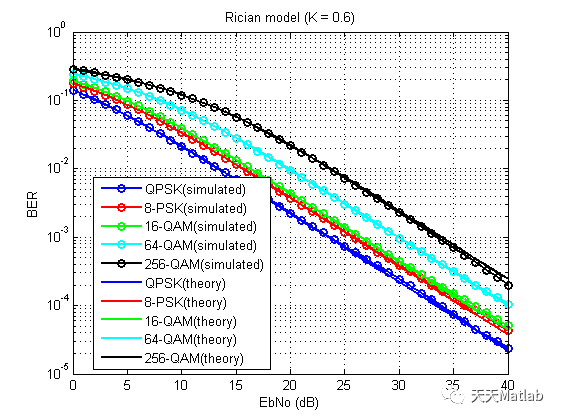60 0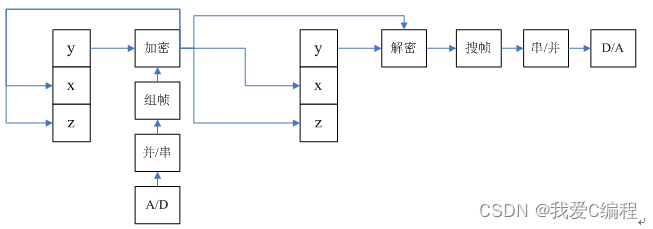m基于Lorenz混沌自同步的混沌数字保密通信系统的FPGA实现,verilog编程实现+MATLAB混沌验证程序
m基于Lorenz混沌自同步的混沌数字保密通信系统的FPGA实现,verilog编程实现+MATLAB混沌验证程序
83 0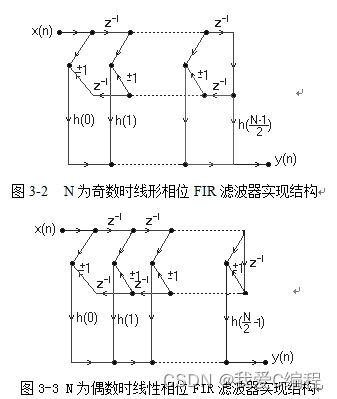m基于Matlab的fir和iir数字滤波器的设计与仿真
m基于Matlab的fir和iir数字滤波器的设计与仿真
205 0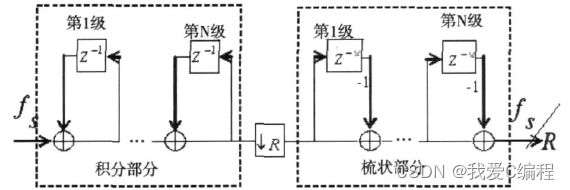m基于FPGA和MATLAB的数字CIC滤波器设计和实现
m基于FPGA和MATLAB的数字CIC滤波器设计和实现
111 0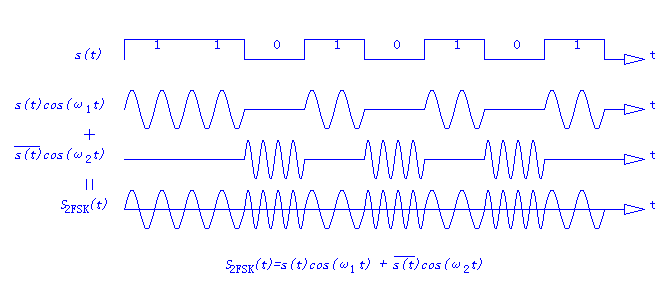99 0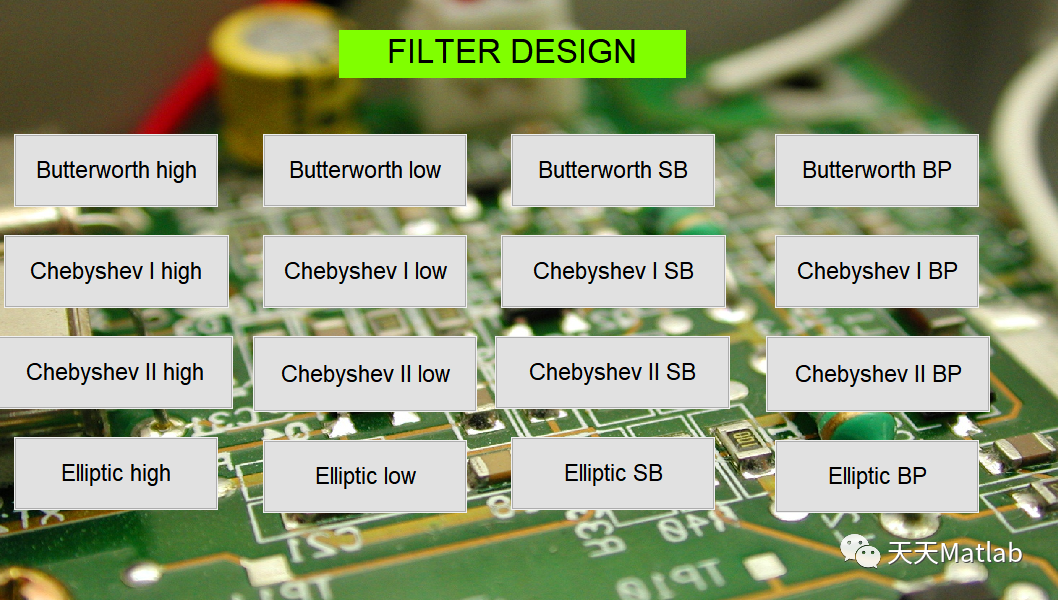【滤波器】基于Matlab设计巴斯 切比雪夫 椭圆 低通高通带通带阻数字滤波器附GUI界面
【滤波器】基于Matlab设计巴斯 切比雪夫 椭圆 低通高通带通带阻数字滤波器附GUI界面
52 0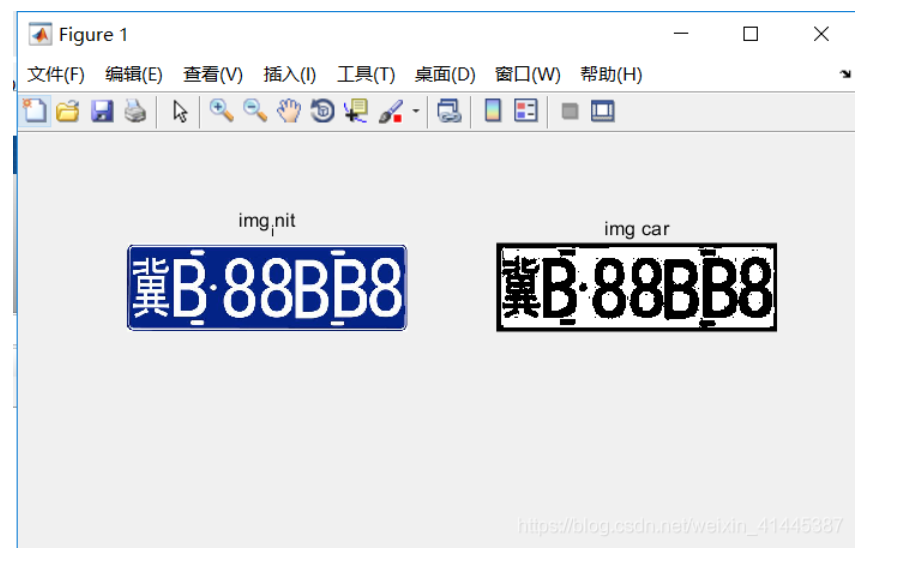44 0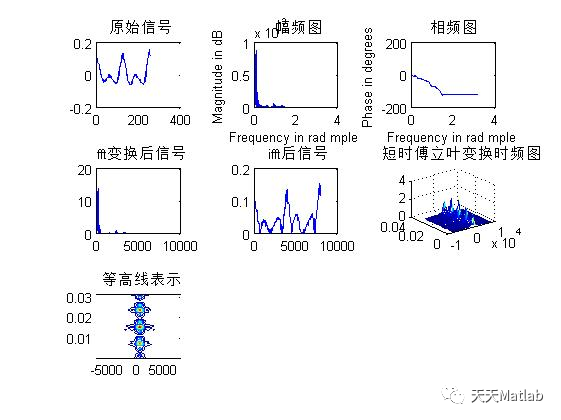【语音识别】基于傅立叶变换和MFCC特征结合DTW算法实现0-9的数字语音识别含Matlab源码
【语音识别】基于傅立叶变换和MFCC特征结合DTW算法实现0-9的数字语音识别含Matlab源码
140 0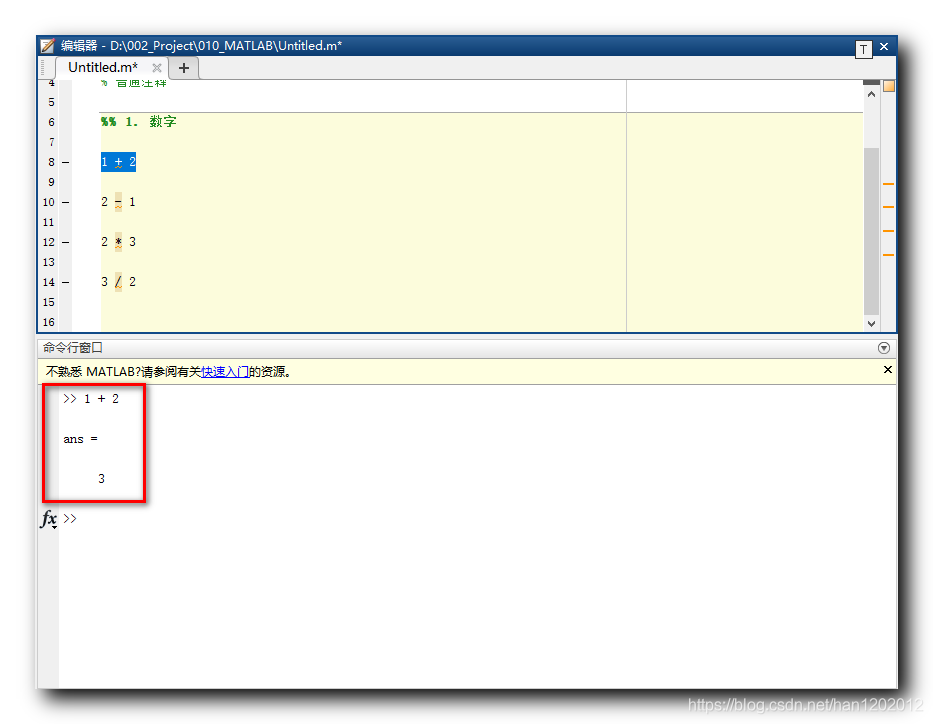【MATLAB】数据类型 ( 执行代码 | 清空命令 | 注释 | 数字 | 字符 | 字符串 )（二）
【MATLAB】数据类型 ( 执行代码 | 清空命令 | 注释 | 数字 | 字符 | 字符串 )（二）
72 0

【算法实战】5. Logistic回归算法446